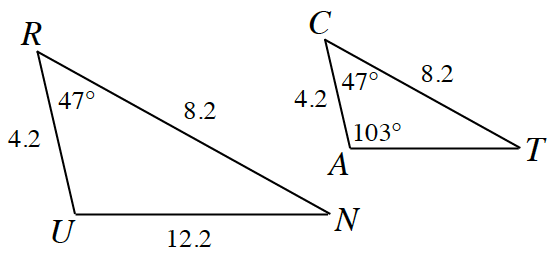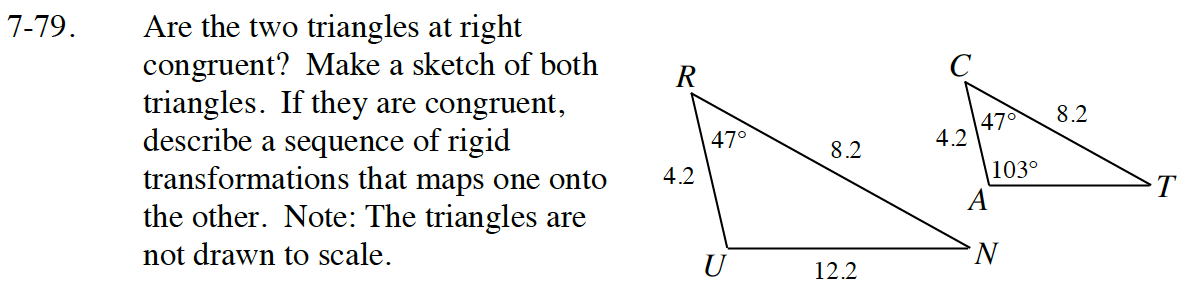Home > CC4 > Chapter 7 > Lesson 7.1.7 > Problem7-79

7-79.Are the two triangles at right congruent? Make a sketch of both triangles. If they are congruent, describe a sequence of rigid transformations that maps one onto the other. Note: The triangles are not drawn to scale. Homework Help ✎Using the three rigid transformations: translation, rotation, and reflection, how can you get ΔRUN to coincide (overlap) ΔCAT?

Read the Math Notes box in Lesson 7.1.7, review the conditions for Triangle Congruence.
Do these triangles have enough congruent parts in the correct order to be considered congruent?